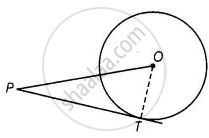# The length of tangent from an external point P on a circle with centre O is always less than OP. - Mathematics

MCQ
True or False

The length of tangent from an external point P on a circle with centre O is always less than OP.

• True

• False

#### Solution

This statement is True.

Explanation:PT is a tangent drawn from external point P. Join OT.

∵ OT ⊥ PT

So, OPT is a right-angled triangle formed.

In right-angled triangle, hypotenuse is always greater than any two of the two sides of the triangle.

∴ OP > PT

or PT < OP

Concept: Number of Tangents from a Point on a Circle
Is there an error in this question or solution?
Chapter 9: Circles - Exercise 9.2 [Page 105]

#### APPEARS IN

NCERT Mathematics Exemplar Class 10
Chapter 9 Circles
Exercise 9.2 | Q 3 | Page 105

Share